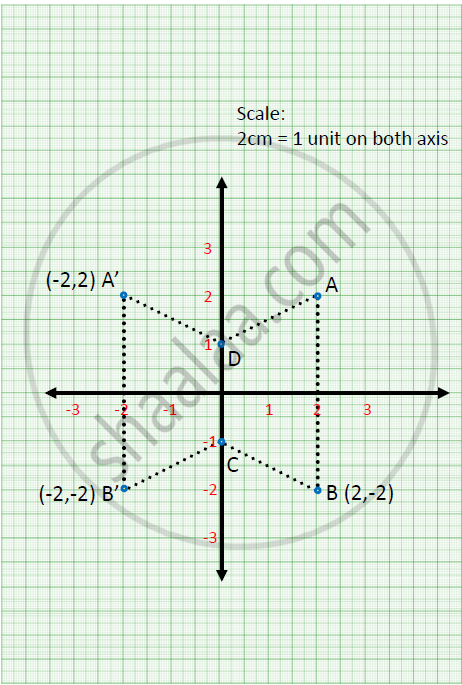Share

# Use Graph Paper for this Question (Take 2 Cm = 1 Unit Along Both X and Y-axis). Abcd is a Quadrilateral Whose Vertices Are A(2, 2), B(2, –2), C(0, –1) and D(0, 1). - ICSE Class 10 - Mathematics

ConceptSimple Applications of All Co-ordinate Geometry.

#### Question

Use graph paper for this question (Take 2 cm = 1 unit along both x and y-axis). ABCD is a quadrilateral whose vertices are A(2, 2), B(2, –2), C(0, –1) and D(0, 1).

1) Reflect quadrilateral ABCD on the y-axis and name it as A'B'CD

2) Write down the coordinates of A' and B'.

3) Name two points which are invariant under the above reflection

4) Name the polygon A'B'CD

#### Solution

(1) and (2)3) D and C are invariant points

4) A’B’CD is a trapezium

Is there an error in this question or solution?

#### APPEARS IN

Solution Use Graph Paper for this Question (Take 2 Cm = 1 Unit Along Both X and Y-axis). Abcd is a Quadrilateral Whose Vertices Are A(2, 2), B(2, –2), C(0, –1) and D(0, 1). Concept: Simple Applications of All Co-ordinate Geometry..
S